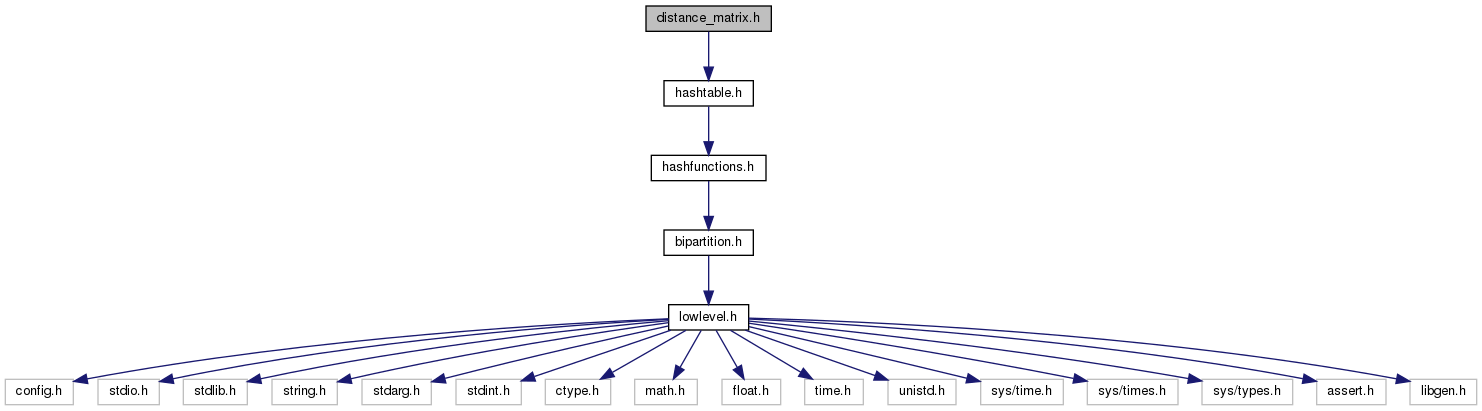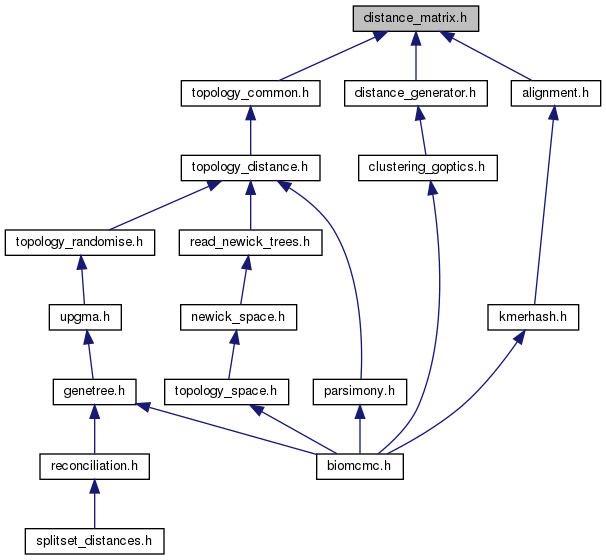biomcmc-lib  0.1 low level library for phylogenetic analysis
distance_matrix.h File Reference

distance matrix, that can be used in alignments and trees, and patristic-distance based species distances More...

`#include "hashtable.h"`
Include dependency graph for distance_matrix.h:This graph shows which files directly or indirectly include this file:Go to the source code of this file.

## Data Structures

struct  distance_matrix_struct

struct  spdist_matrix_struct

## Typedefs

typedef struct distance_matrix_structdistance_matrix

typedef struct spdist_matrix_structspdist_matrix

## Functions

distance_matrix new_distance_matrix (int nseqs)
creates new matrix of pairwise distances

void zero_lower_distance_matrix (distance_matrix dist)
specially in gene/sptree distance methods (GLASS, STEAC, etc.) lower is used for means and upper for min. This function resets matrix elements

void transpose_distance_matrix (distance_matrix dist)
invert lower and upper diagonals of matrix (since some functions like upgma expect upper, etc.)

void del_distance_matrix (distance_matrix dist)
releases memory allocated to distance_matrix (this structure has no smart ref_counter)

spdist_matrix new_spdist_matrix (int n_species)

void zero_all_spdist_matrix (spdist_matrix dist)

void finalise_spdist_matrix (spdist_matrix dist)

void finalise_spdist_matrix_with_rescaling (spdist_matrix dist, double scale)

void complete_missing_spdist_from_global_spdist (spdist_matrix local, spdist_matrix global)

void copy_spdist_matrix_to_distance_matrix_upper (spdist_matrix spd, distance_matrix dist, bool use_means)

void del_spdist_matrix (spdist_matrix dist)

void fill_species_dists_from_gene_dists (distance_matrix spdist, distance_matrix gendist, int *sp_id, bool use_upper_gene)
updates distances between species based on genes and gene-to-species mapping, with min on upper and mean on lower diagonal

void update_species_dists_from_spdist (distance_matrix global, distance_matrix local, int *spexist)
update global (over loci) species distances besed on local (within locus) species distances

int prepare_spdistmatrix_from_gene_species_map (spdist_matrix spdist, int *sp_id, int n_sp_id)

void fill_spdistmatrix_from_gene_dists (spdist_matrix spdist, distance_matrix gendist, int *sp_id, bool use_upper_gene)

void fill_spdistmatrix_from_gene_dist_vector (spdist_matrix spdist, double *gdist, int n_gdist, int *sp_id)
initialise spdist_matrix with patristic distances from gdist vector of size n_gdist (1D)

void update_spdistmatrix_from_spdistmatrix (spdist_matrix global, spdist_matrix local)

## Detailed Description

distance matrix, that can be used in alignments and trees, and patristic-distance based species distances

These functions don't know about trees/topologies: topology_common.c creates the actual patristic distances, and downstream software or genetree.h should decide how to use this information

## ◆ zero_all_spdist_matrix()

 void zero_all_spdist_matrix ( spdist_matrix dist )

zero both mean[] and min[] since we only look at average (never min) across loci